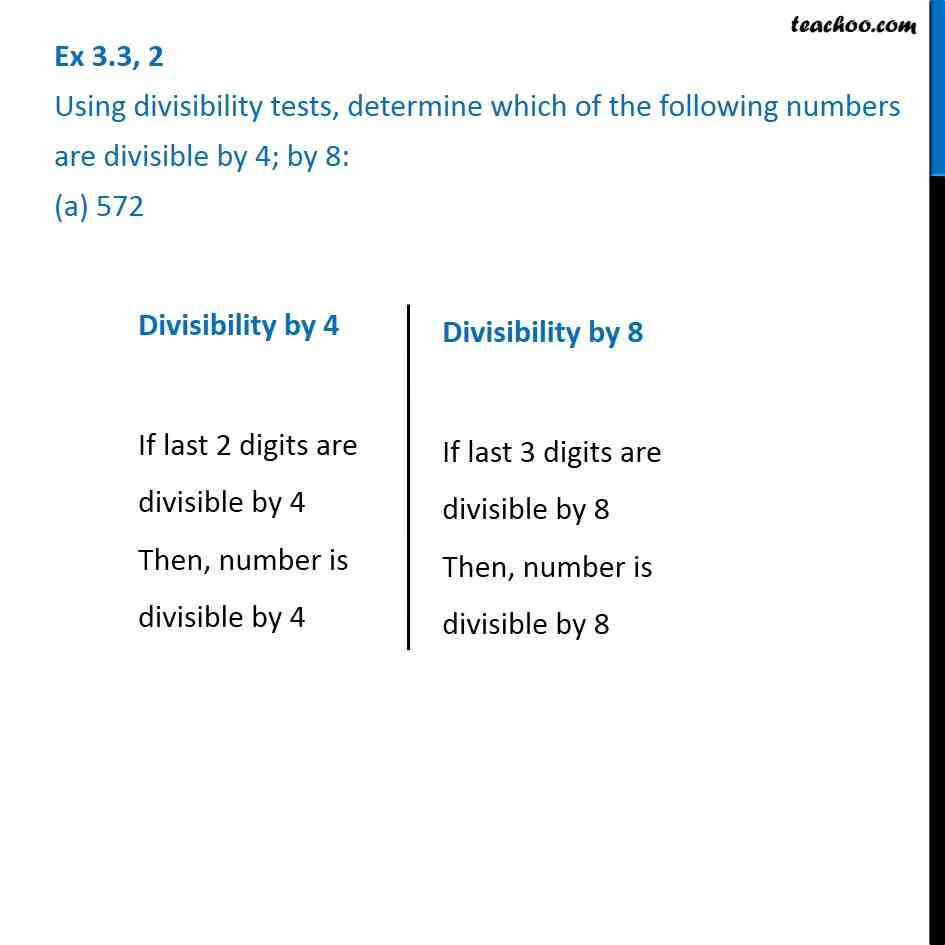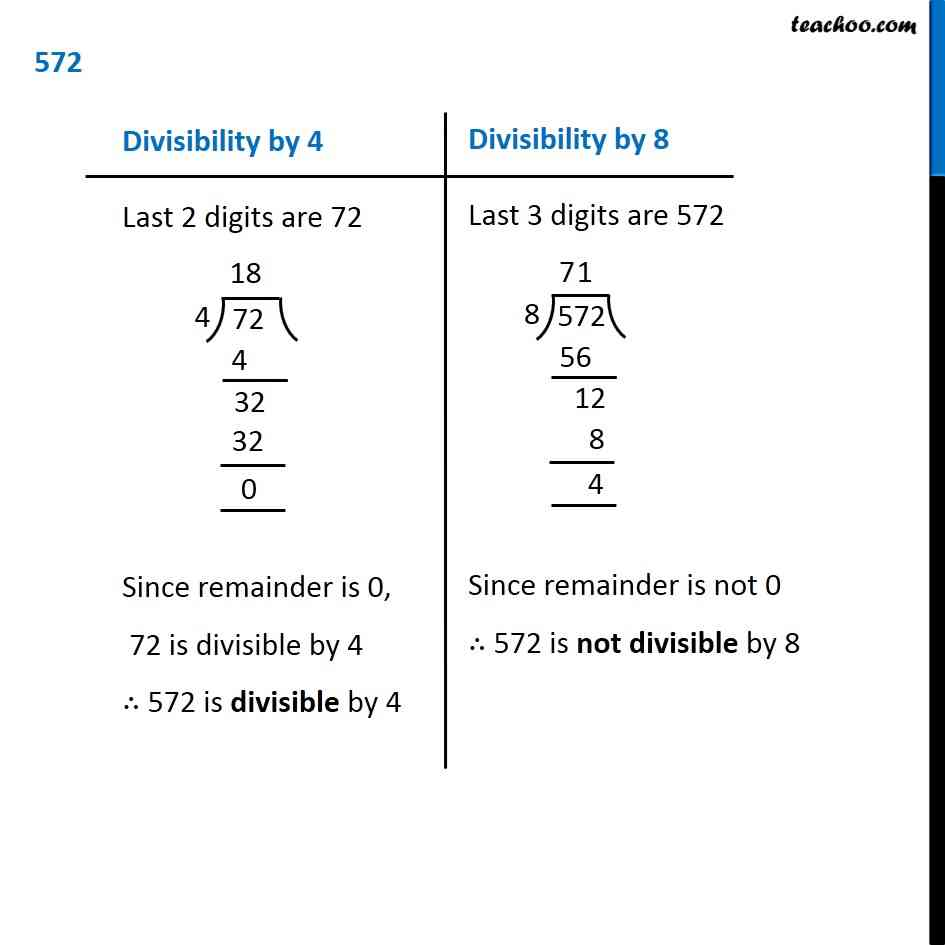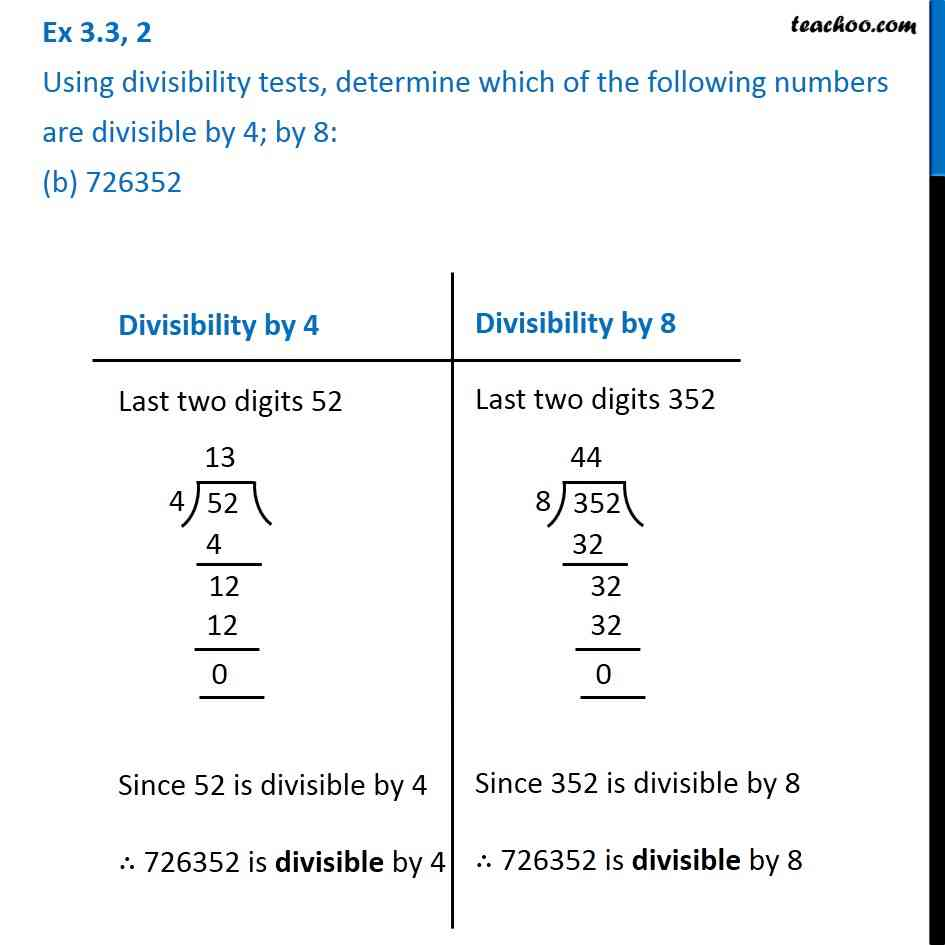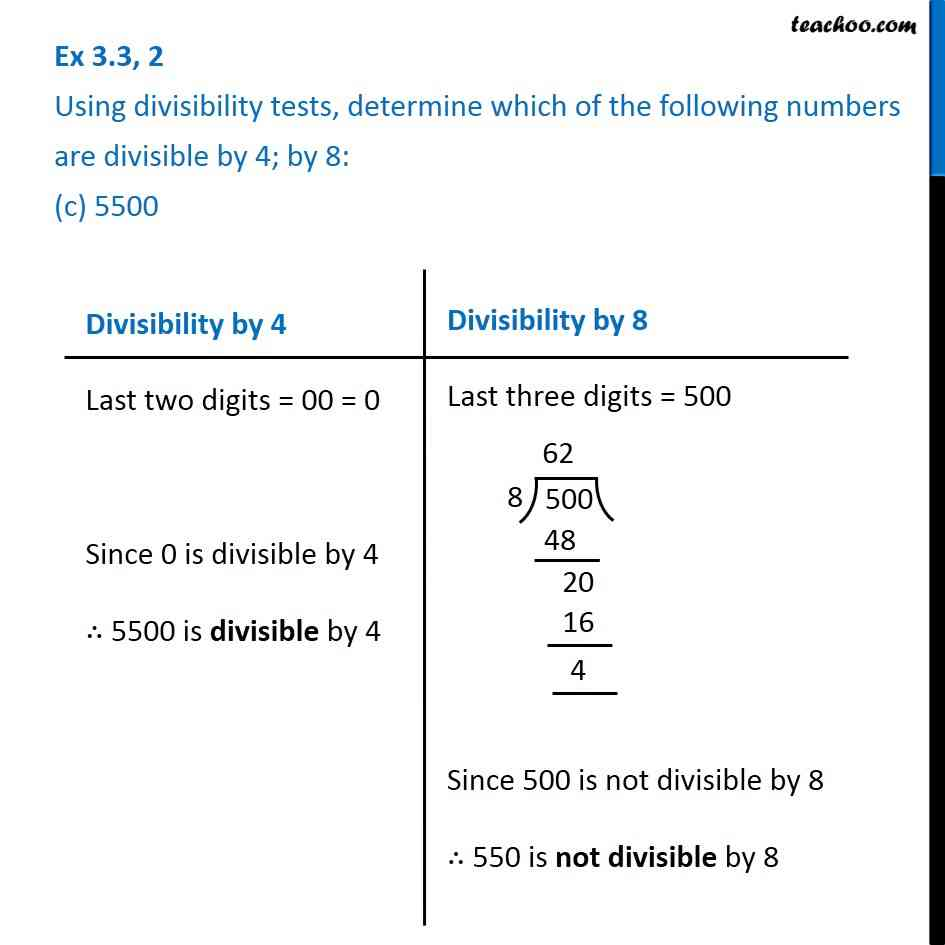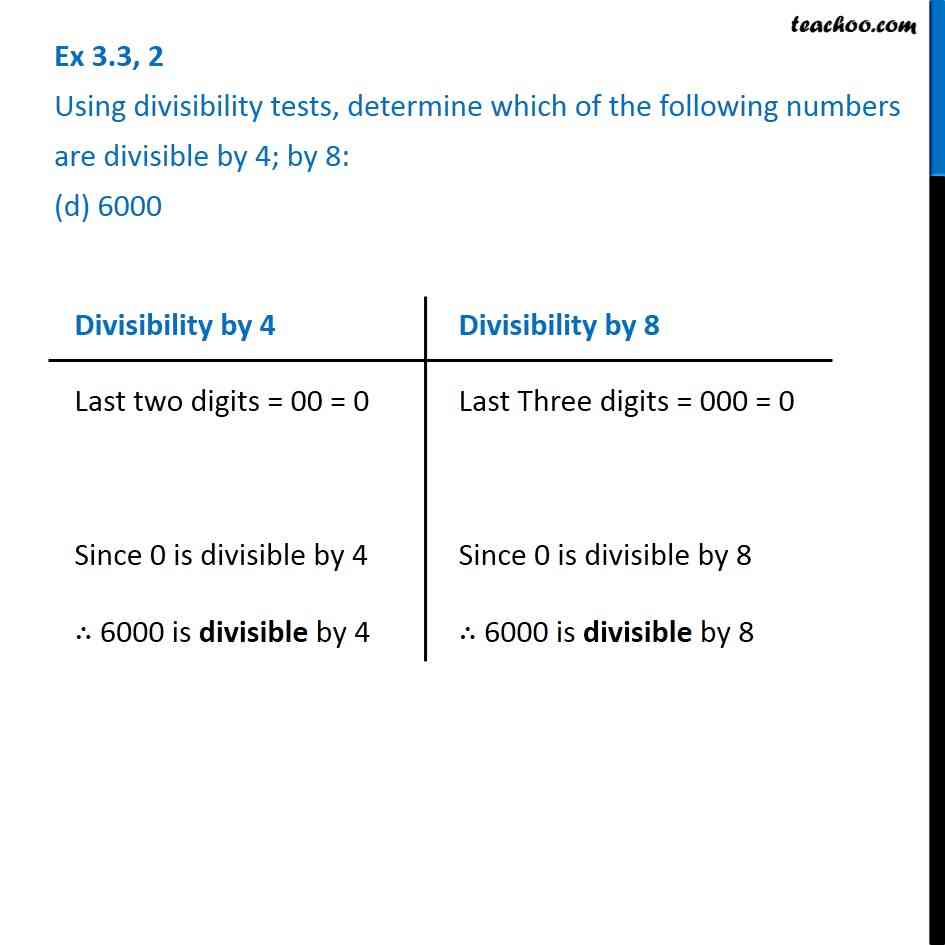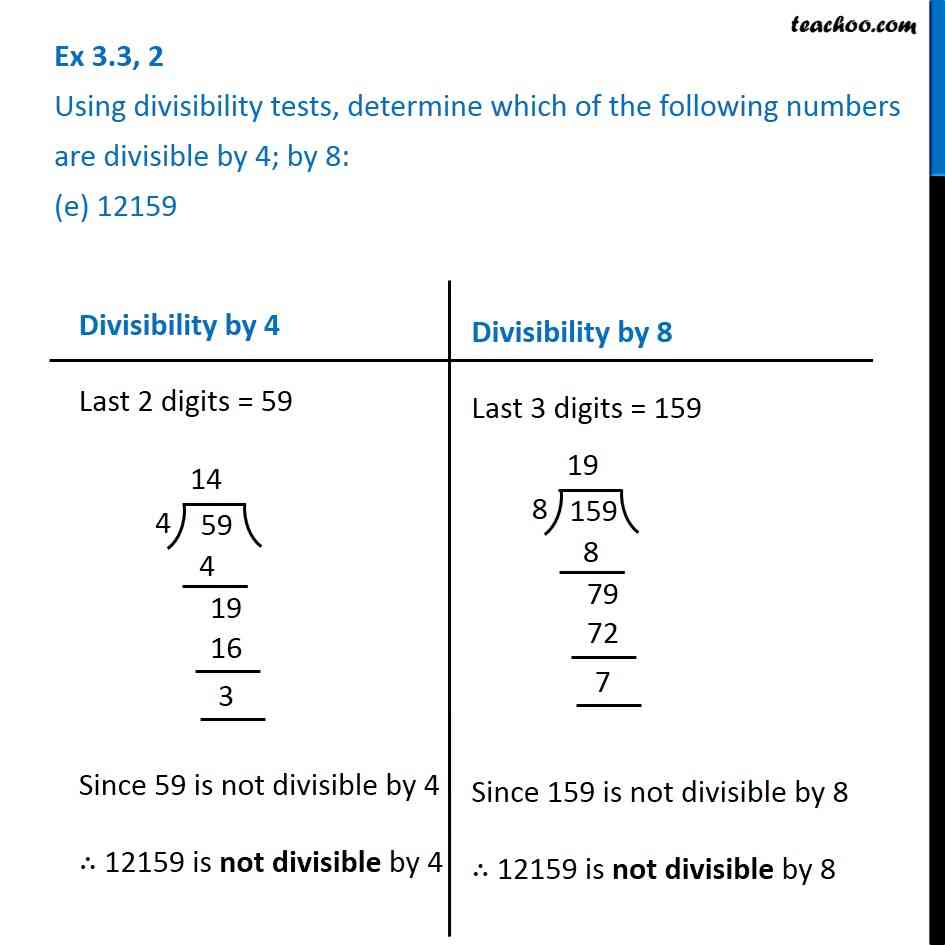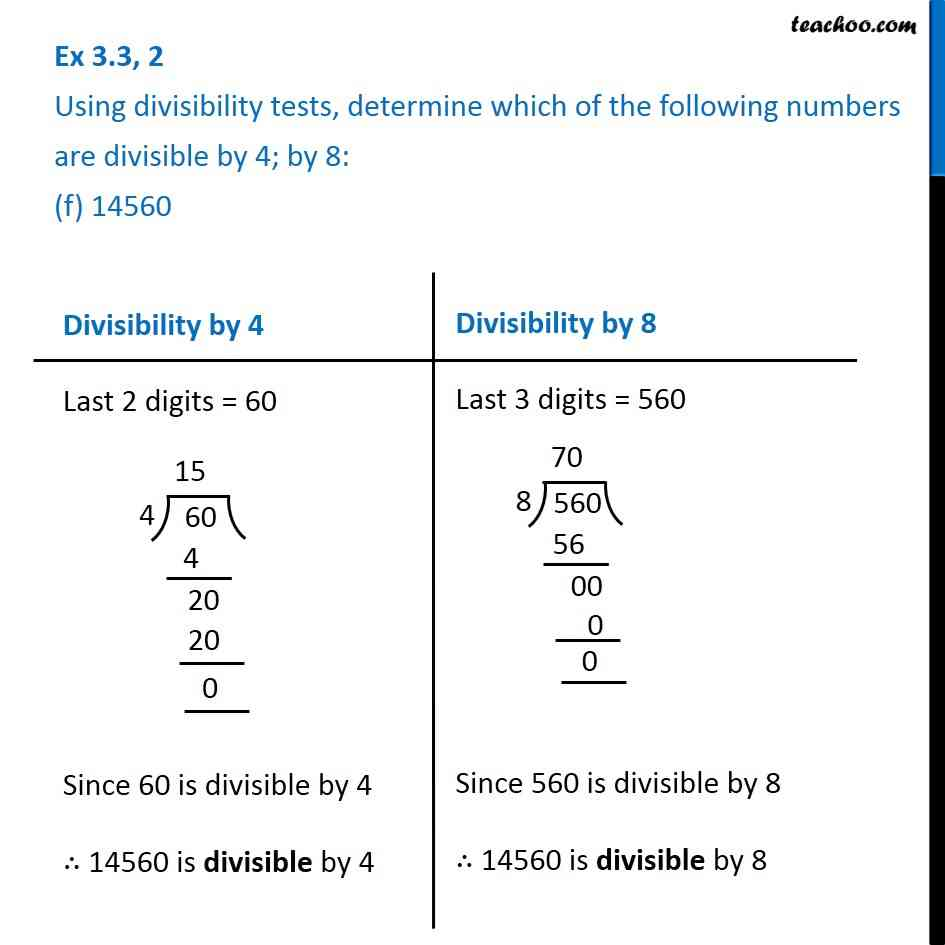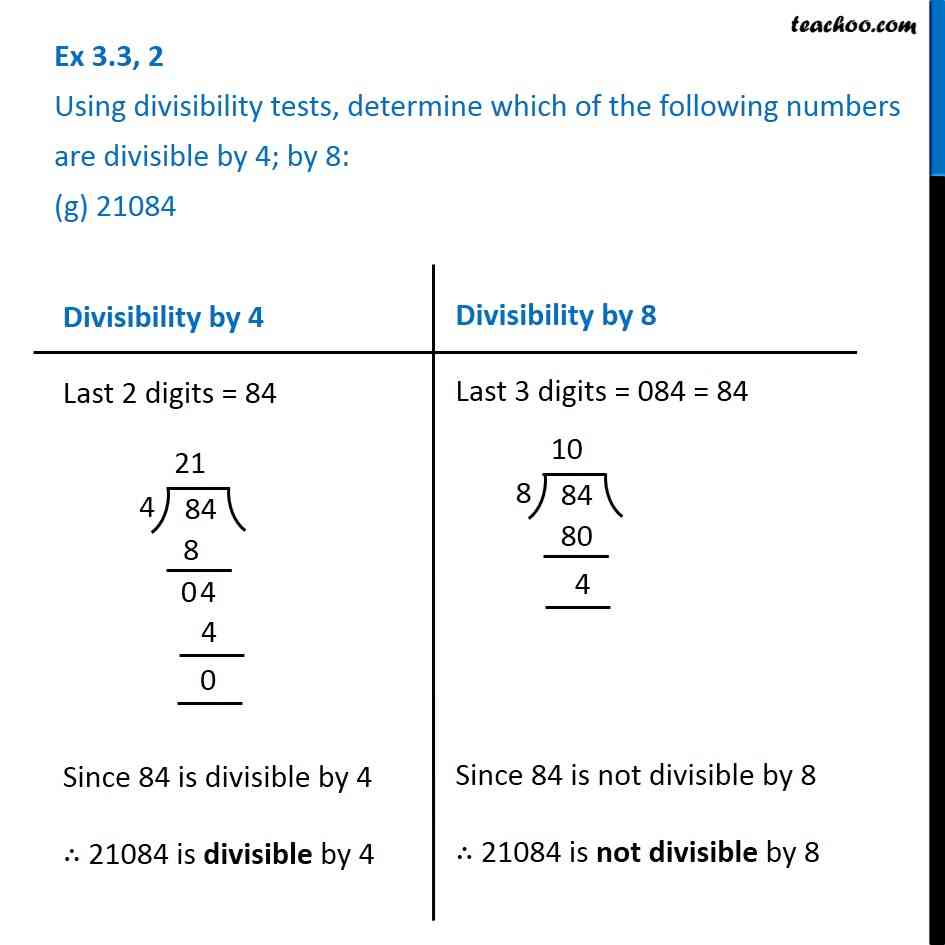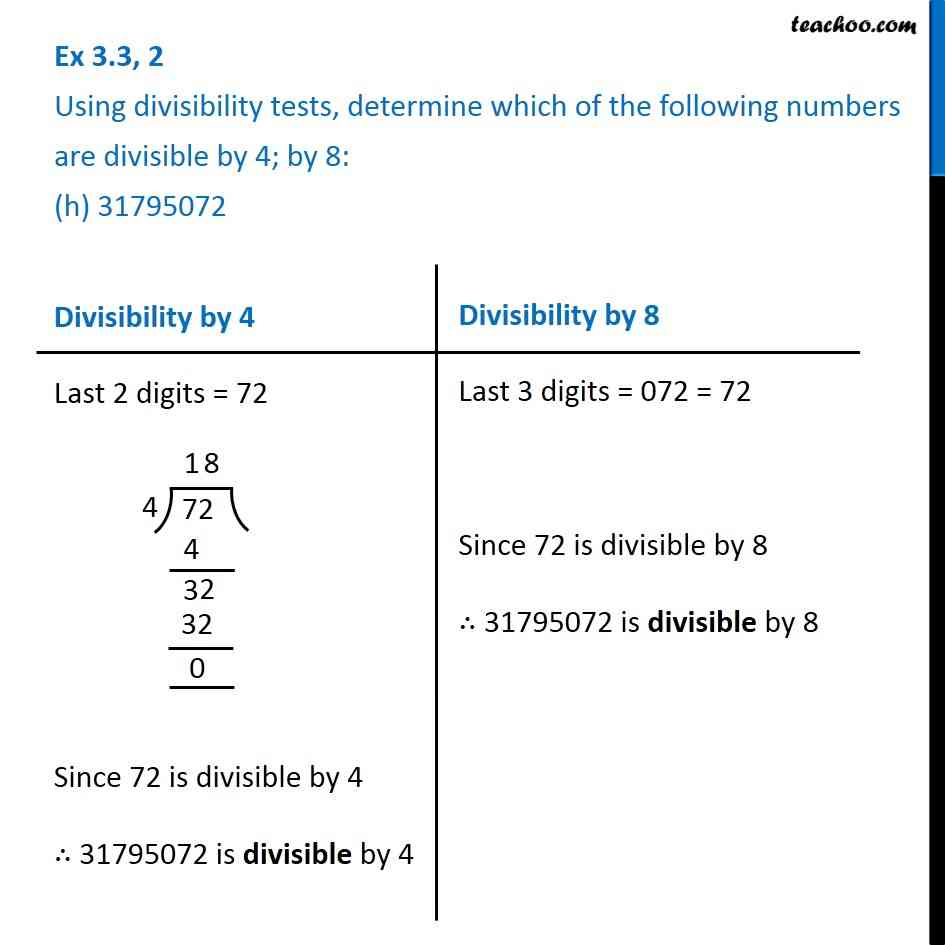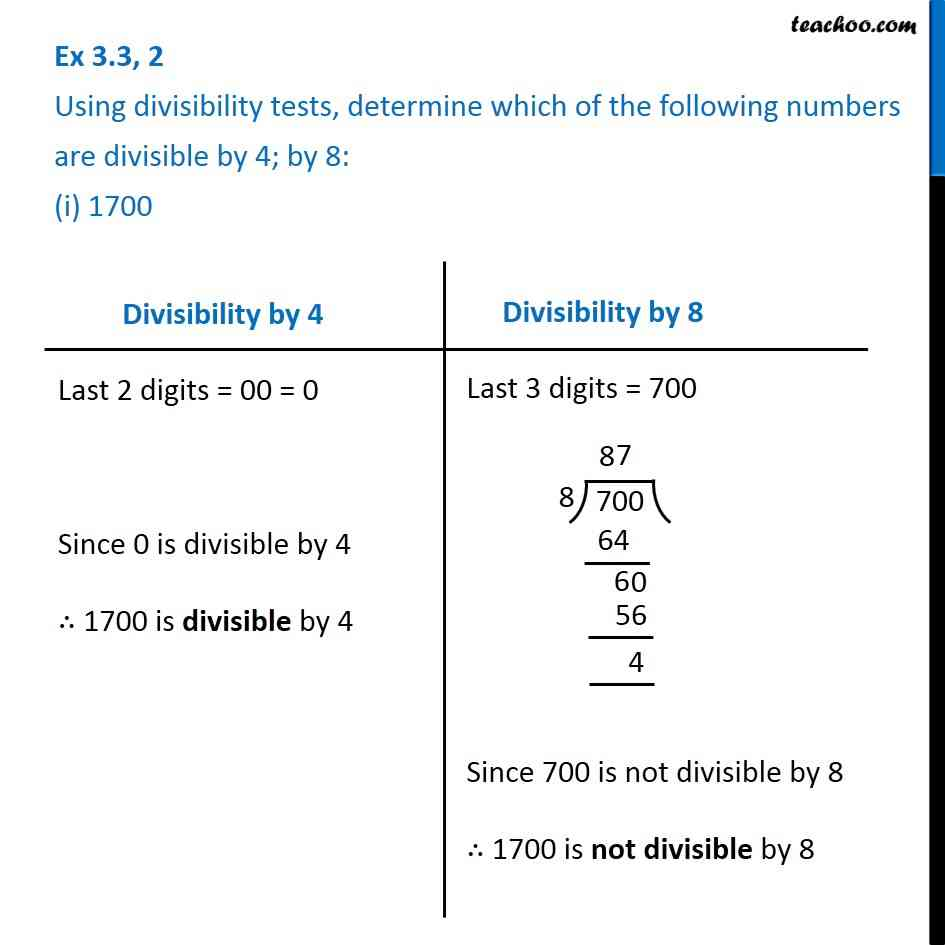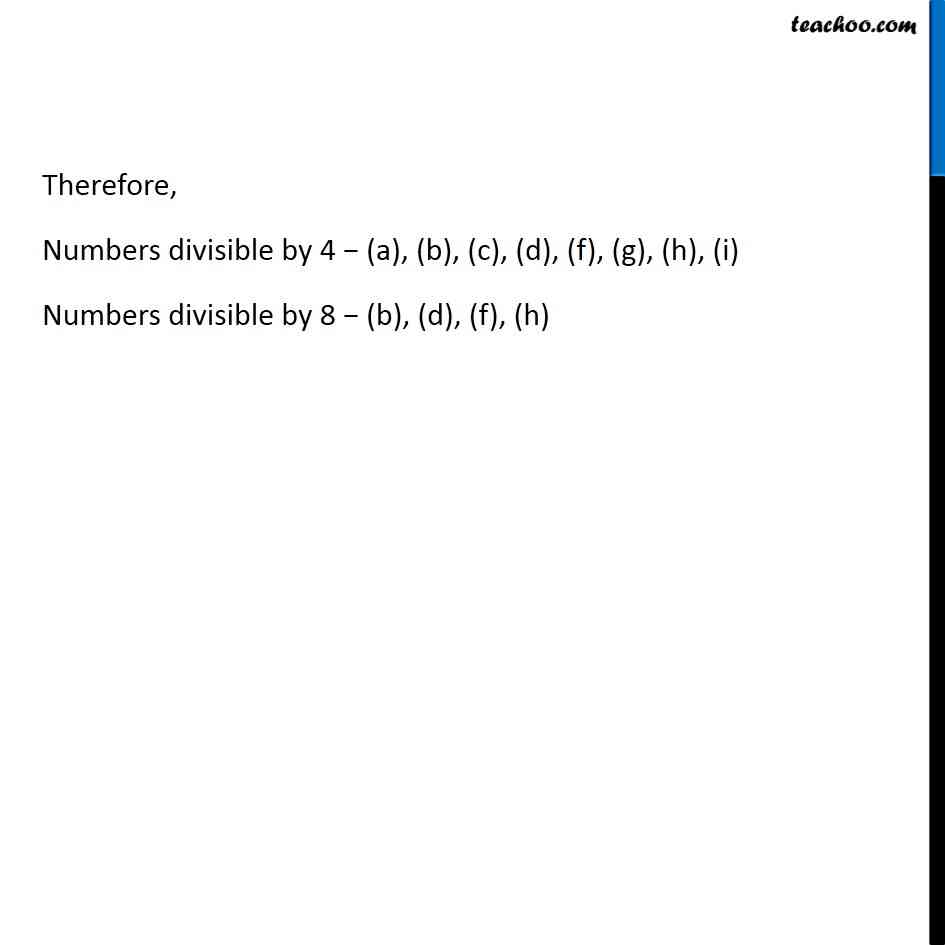1. Chapter 3 Class 6 Playing with Numbers
2. Serial order wise
3. Ex 3.3

Transcript

Ex 3.3, 2 Using divisibility tests, determine which of the following numbers are divisible by 4; by 8: (a) 572 Divisibility by 4 If last 2 digits are divisible by 4 Then, number is divisible by 4 Divisibility by 8 If last 3 digits are divisible by 8 Then, number is divisible by 8 Divisibility by 8 If last 3 digits are divisible by 8 Then, number is divisible by 8 Divisibility by 4 Last 2 digits are 72 Since remainder is 0, 72 is divisible by 4 ∴ 572 is divisible by 4 Divisibility by 8 Last 3 digits are 572 Since remainder is not 0 ∴ 572 is not divisible by 8 Ex 3.3, 2 Using divisibility tests, determine which of the following numbers are divisible by 4; by 8: (b) 726352 Divisibility by 4 Last two digits 52 Since 52 is divisible by 4 ∴ 726352 is divisible by 4 Divisibility by 8 Last two digits 352 Since 352 is divisible by 8 ∴ 726352 is divisible by 8 Divisibility by 8 Last two digits 352 Since 352 is divisible by 8 ∴ 726352 is divisible by 8 Ex 3.3, 2 Using divisibility tests, determine which of the following numbers are divisible by 4; by 8: (c) 5500 Divisibility by 4 Last two digits = 00 = 0 Since 0 is divisible by 4 ∴ 5500 is divisible by 4 Divisibility by 8 Last three digits = 500 Since 500 is not divisible by 8 ∴ 550 is not divisible by 8 Ex 3.3, 2 Using divisibility tests, determine which of the following numbers are divisible by 4; by 8: (d) 6000 Divisibility by 4 Last two digits = 00 = 0 Since 0 is divisible by 4 ∴ 6000 is divisible by 4 Divisibility by 8 Last Three digits = 000 = 0 Since 0 is divisible by 8 ∴ 6000 is divisible by 8 Divisibility by 8 Last Three digits = 000 = 0 Since 0 is divisible by 8 ∴ 6000 is divisible by 8 Ex 3.3, 2 Using divisibility tests, determine which of the following numbers are divisible by 4; by 8: (e) 12159 Divisibility by 4 Last 2 digits = 59 Since 59 is not divisible by 4 ∴ 12159 is not divisible by 4 Divisibility by 8 Last 3 digits = 159 Since 159 is not divisible by 8 ∴ 12159 is not divisible by 8 Ex 3.3, 2 Using divisibility tests, determine which of the following numbers are divisible by 4; by 8: (f) 14560 Divisibility by 4 Last 2 digits = 60 Since 60 is divisible by 4 ∴ 14560 is divisible by 4 Divisibility by 8 Last 3 digits = 560 Since 560 is divisible by 8 ∴ 14560 is divisible by 8 Ex 3.3, 2 Using divisibility tests, determine which of the following numbers are divisible by 4; by 8: (g) 21084 Divisibility by 4 Last 2 digits = 84 Since 84 is divisible by 4 ∴ 21084 is divisible by 4 Divisibility by 8 Last 3 digits = 084 = 84 Since 84 is not divisible by 8 ∴ 21084 is not divisible by 8 Ex 3.3, 2 Using divisibility tests, determine which of the following numbers are divisible by 4; by 8: (h) 31795072 Divisibility by 4 Last 2 digits = 72 Since 72 is divisible by 4 ∴ 31795072 is divisible by 4 Divisibility by 8 Last 3 digits = 072 = 72 Since 72 is divisible by 8 ∴ 31795072 is divisible by 8 Ex 3.3, 2 Using divisibility tests, determine which of the following numbers are divisible by 4; by 8: (i) 1700 Divisibility by 4 Last 2 digits = 00 = 0 Since 0 is divisible by 4 ∴ 1700 is divisible by 4 Divisibility by 8 Last 3 digits = 700 Since 700 is not divisible by 8 ∴ 1700 is not divisible by 8 Ex 3.3, 2 Using divisibility tests, determine which of the following numbers are divisible by 4; by 8: (j) 2150 Divisibility by 4 Last 2 digits = 50 Since 50 is not divisible by 4 ∴ 2150 is not divisible by 4 Divisibility by 8 Last 3 digits = 150 Since 150 is not divisible by 8 ∴ 2150 is not divisible by 8 Therefore, Numbers divisible by 4 − (a), (b), (c), (d), (f), (g), (h), (i) Numbers divisible by 8 − (b), (d), (f), (h)

Ex 3.3

About the AuthorDavneet Singh
Davneet Singh is a graduate from Indian Institute of Technology, Kanpur. He has been teaching from the past 10 years. He provides courses for Maths and Science at Teachoo.